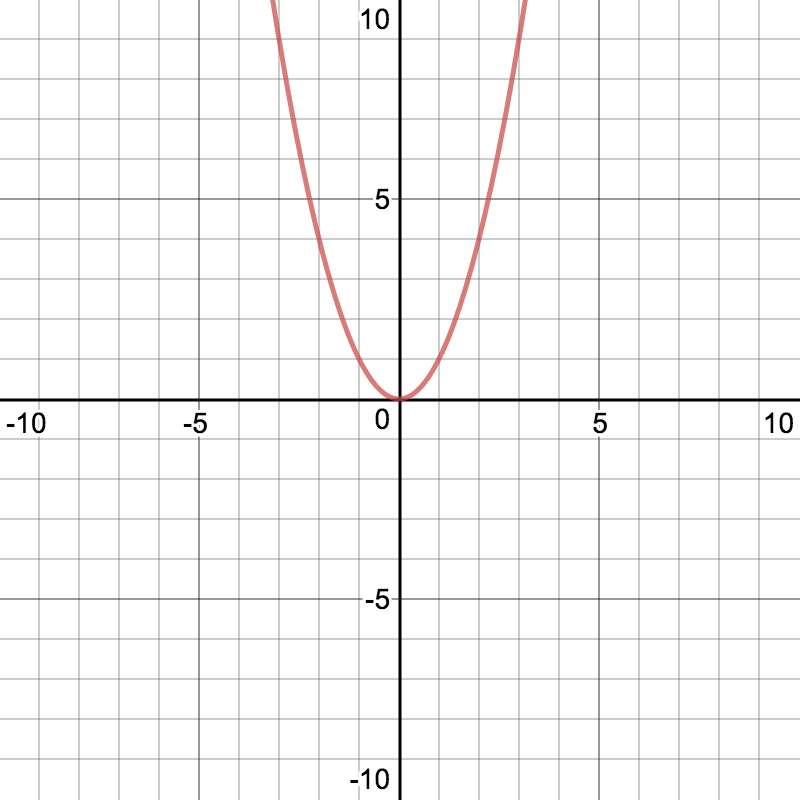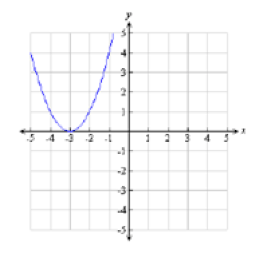# FNGR 2 | Lesson 1 | Making ConnectionsMatch the graph to the table of values and equation that it represents. Graphs are created using Desmos.com.

1.

 x -2 -1 0 1 y 4 -2 -4 -2
2.3. $$y=x^2$$
4.5.

 x -1 0 1 2 y 4 9 16 25
6. $$y=2x^2 -4$$
7. $$y=x^2-2x+3$$ 8.

 x -2 -1 0 1 y 4 1 0 1
9.10.11. $$y=(x+3)^2$$ 12.

 x -1 0 1 2 y 7 3 0 3